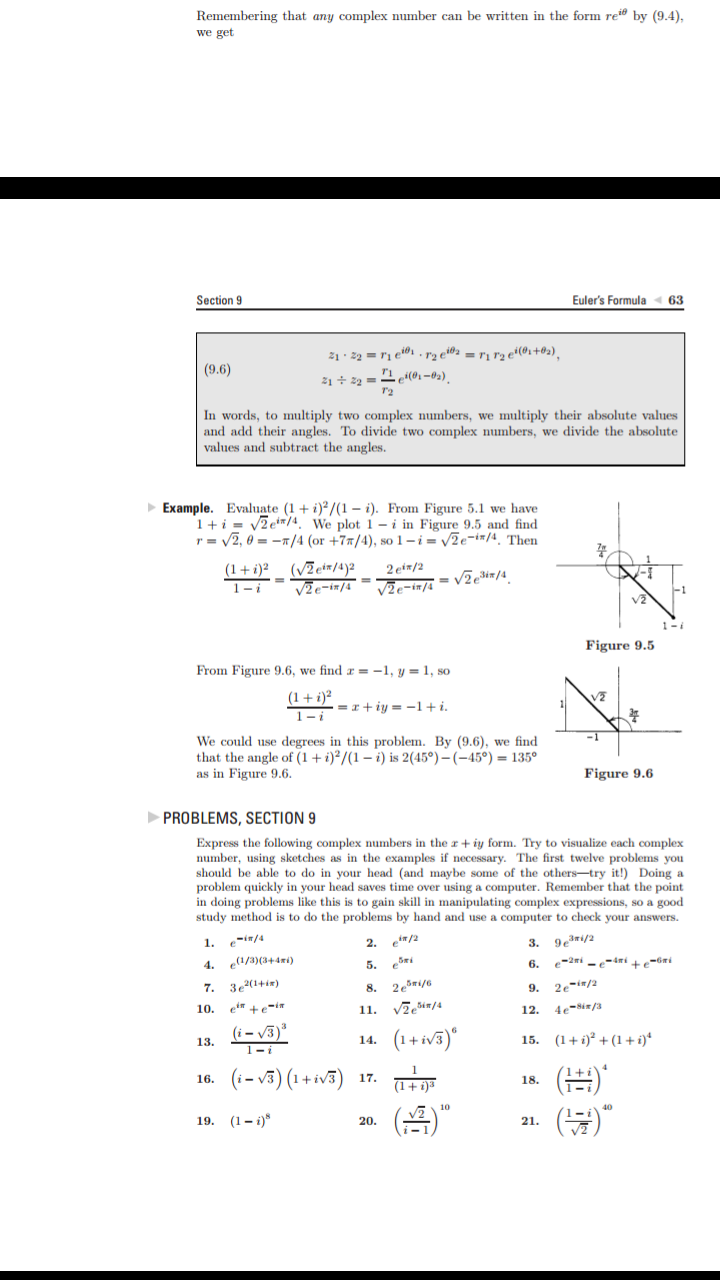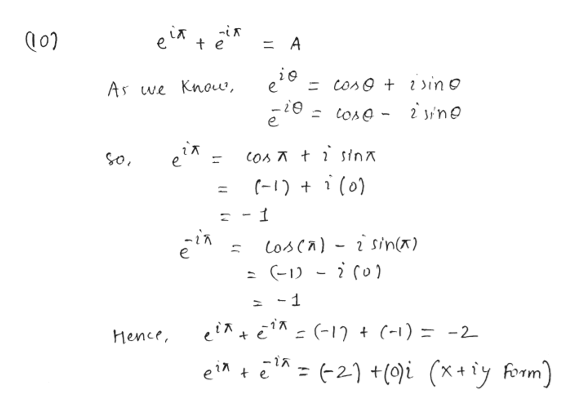# Remembering that any complex number can be written in the form re" by (9.4),we getSection 9Euler's Formula632 e2 = ri r2 e4(02+@2),e21 22(9.6)In words, to multiply two complex numbers, we multiply their absolute valuesand add their angles. To divide two complex numbers, we divide the absolutevalues and subtract the anglesExample. Evaluate (1 + i)?/(1 - i). From Figure 5.1 we have1 2et/4. We plot 1 - i in Figure 9.5 and findr2, 0=/4 (or +7/4), so 1-i= /2e-i#/4. Then(VEetr/a)2V2e-i7/4=J%-in/s = /2e3i#/42 eir/2(1+i)21-1Figure 9.5From Figure 9.6, we find =-1, y = 1, so(1i)21-1=riy-1+iWe could use degrees in this problem. By (9.6), we findthat the angle of (1 i)2/(1-i) is 2(45°) - (-45°) 135°as in Figure 9.6Figure 9.6PROBLEMS, SECTION 9Express the following complex numbers in the r + iy form. Try to visualize each complexnumber, using sketches as in the examples if necessary. The first twelve problems youshould be able to do in your head (and maybe some of the others-try it!) Doing aproblem quickly in your head saves time over using a computer. Remember that the pointin doing problems like this is to gain skill in manipulating complex expressions, so a goodstudy method is to do the problems by hand and use a computer to check your answers.3. 93ri/2e-2i -4mi -2. ei/21. ei/4(a/3)(344mi4.6.5.7. 3e2(1+i2esri/69.2e-i/2/44e-Sin/310.11.12.(i)1-i(1+ W)15. (1 (1 i)*14.13.((-)(1+v)17.16()19. (1-)2120.

Question
1 viewshelp_outlineImage TranscriptioncloseRemembering that any complex number can be written in the form re" by (9.4), we get Section 9 Euler's Formula 63 2 e2 = ri r2 e4(02+@2), e 21 22 (9.6) In words, to multiply two complex numbers, we multiply their absolute values and add their angles. To divide two complex numbers, we divide the absolute values and subtract the angles Example. Evaluate (1 + i)?/(1 - i). From Figure 5.1 we have 1 2et/4. We plot 1 - i in Figure 9.5 and find r2, 0=/4 (or +7/4), so 1-i= /2e-i#/4. Then (VEetr/a)2 V2e-i7/4=J%-in/s = /2e3i#/4 2 eir/2 (1+i)2 1-1 Figure 9.5 From Figure 9.6, we find =-1, y = 1, so (1i)2 1-1 =riy-1+i We could use degrees in this problem. By (9.6), we find that the angle of (1 i)2/(1-i) is 2(45°) - (-45°) 135° as in Figure 9.6 Figure 9.6 PROBLEMS, SECTION 9 Express the following complex numbers in the r + iy form. Try to visualize each complex number, using sketches as in the examples if necessary. The first twelve problems you should be able to do in your head (and maybe some of the others-try it!) Doing a problem quickly in your head saves time over using a computer. Remember that the point in doing problems like this is to gain skill in manipulating complex expressions, so a good study method is to do the problems by hand and use a computer to check your answers. 3. 93ri/2 e-2i -4mi - 2. ei/2 1. ei/4 (a/3)(344mi 4. 6. 5. 7. 3e2(1+i 2esri/6 9. 2e-i/2 /4 4e-Sin/3 10. 11. 12. (i) 1-i (1+ W) 15. (1 (1 i)* 14. 13. ( (-)(1+v) 17. 16 () 19. (1-) 21 20. fullscreen
check_circle

Step 1

Solving part 10:help_outlineImage Transcriptionclose= A t e i sin o tosg As we Knou, COs A tsin So (o) (-1) - - 1 Los ca) sin(x) 1 ei (12 + (-1) = -2 Hence ein e1^= (2) +(9)i (xiy form) 2) +0i (xiy fullscreen
Step 2

Solving question...

### Want to see the full answer?

See Solution

#### Want to see this answer and more?

Solutions are written by subject experts who are available 24/7. Questions are typically answered within 1 hour.*

See Solution
*Response times may vary by subject and question.
Tagged in

### Physics